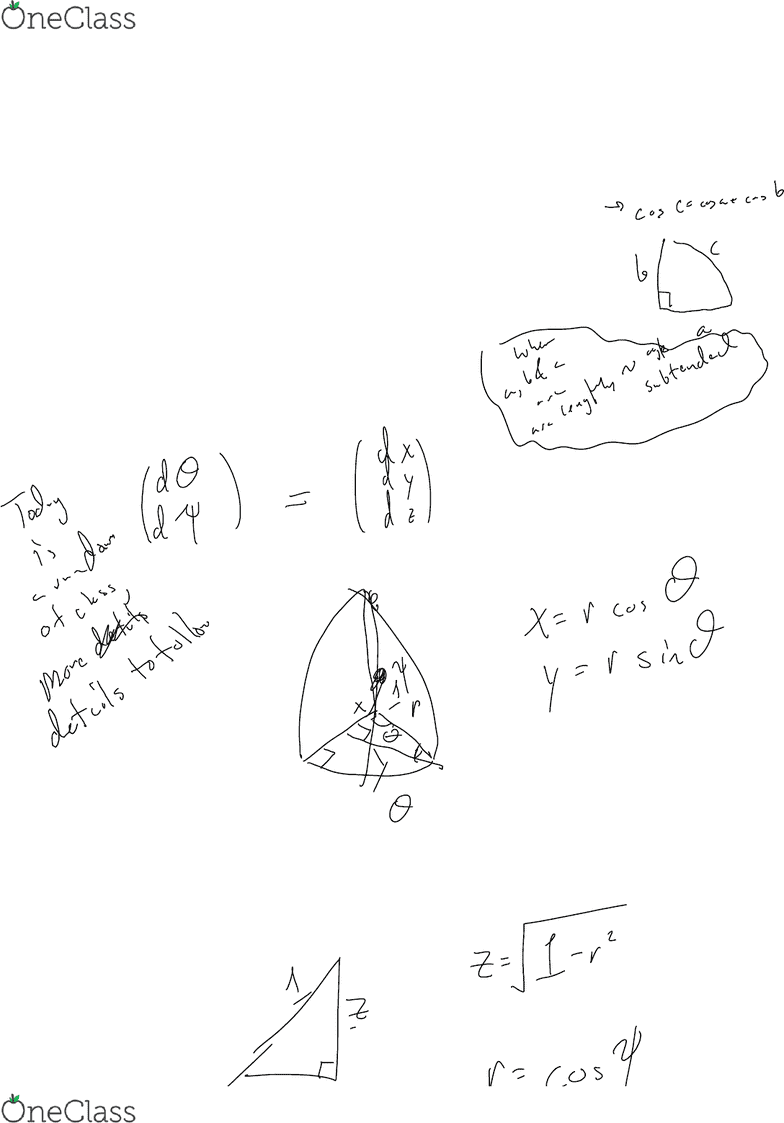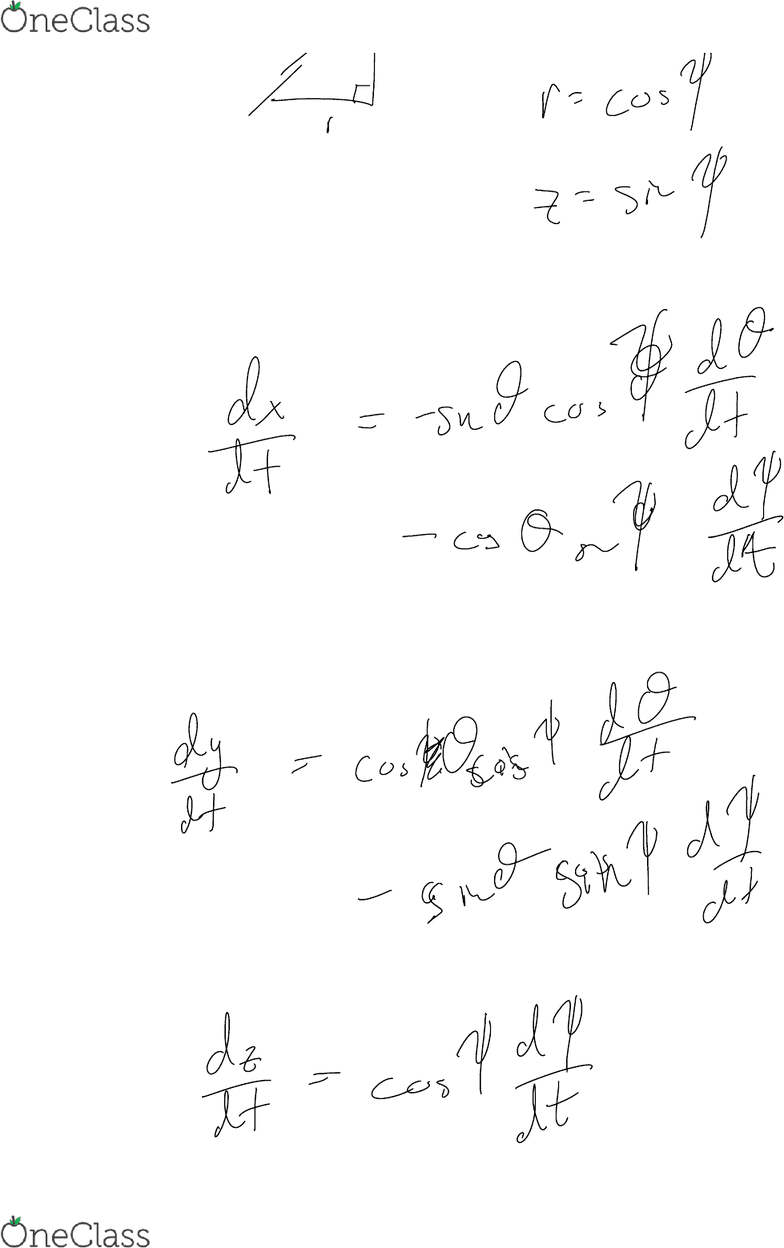# MATH V3386- Midterm Exam Guide - Comprehensive Notes for the exam ( 63 pages long!)

79 views63 pagesColumbia
MATH V3386
MIDTERM EXAM
STUDY GUIDE
Unlock document

This preview shows pages 1-3 of the document.
Unlock all 63 pages and 3 million more documents.Unlock document

This preview shows pages 1-3 of the document.
Unlock all 63 pages and 3 million more documents.Differential Geometry --Gemomety comes from geos--> soil land or earth, metricein--> to rule
So geometry means to rule the world, kinda
The first deviation from plane geometry was geometry on a curved surface
Now the question is where do the derivates come in?
dx and dy (in a plane)
So we take a tangent and define its parts as
Starting from the center of a sphere, we pick
And
ψ=latitude
Some use
φ=π/2-ψ=co-latitude
Tangent vector has coordiantes
Introduction and Outline
Tuesday, September 5, 2017
11:42 AM
September 5th Page 1
find more resources at oneclass.com
find more resources at oneclass.com
Unlock document

This preview shows pages 1-3 of the document.
Unlock all 63 pages and 3 million more documents.September 5th Page 2
find more resources at oneclass.com
find more resources at oneclass.com
Unlock document

This preview shows pages 1-3 of the document.
Unlock all 63 pages and 3 million more documents.

## Document Summary

Differential geometry --gemomety comes from geos--> soil land or earth, metricein--> to rule. So geometry means to rule the world, kinda. The first deviation from plane geometry was geometry on a curved surface. So we take a tangent and define its parts as dx and dy (in a plane) Starting from the center of a sphere, we pick. A euclidean set is a sub set of a euclidean space. An implicit representation of a set will tell you if a point lies in a set, but not which points explicitly. A map f:a->b from a to b is a rule which for each x in a assigns the point y=f(x) in b. F is one-to-one (injective) if f(x1)=f(x2) iff x1=x2. F is onto (surjective) if for every y in b, there exists at least one x in a such that f(x)=y. Vector valued functions map 1d on many dimensions. Function of vector variable 2+d->1d (also functions of several variables)